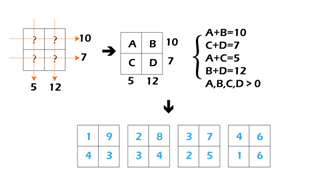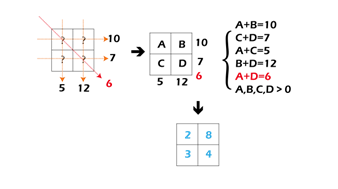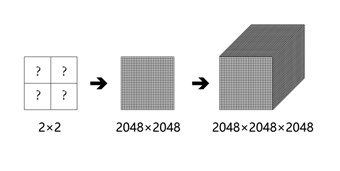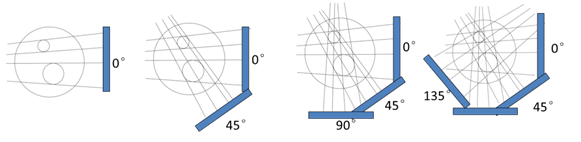Stock code:839222
Hotline:+86-22-24984836

#### CT 3D Reconstruction

The full name of CT is Computed Tomography. Through the relative movement of X-ray source, sample and detector, a series of perspective projections of samples (usually called DR, Digital Radiography) are collected from different angles, and then with the three-dimensional reconstruction, a series of virtual sectioning images inside the sample can be obtained to complete the three-dimensional structure reconstruction.

The core of CT technology is the theory of image rebuilding from the projection data. Its essence is to calculate the attenuation coefficient of each point on the imaging plane from the projection data (DR) obtained by scanning.

The principle of back projection rebuilding is briefly explained below.

I. Algebraic form

Suppose there is a 2 × 2 two-dimensional plane grid as shown in the figure below, and there is an unknown natural number in each grid. We need to calculate four unknowns A, B, C and D according to the sum of projections in different directions.

First, we have obtained the projection information in two directions at 0 and 90 degrees, established an equation set, and found that there are 4 possible solutions for the unknown.Obviously, the above results are far from the truth, which reminds us that the projection data with only 0 and 90 degrees are not enough, and we add another 45 degrees (as shown in the figure below). And then a unique solution can be obtained.The above process is a simple 2 × 2 two-dimensional plane grid and so forth. As shown in the figure below, the actual CT rebuilding is the reverse process of 3D grid with huge amount of data.II. Geometrical form

The process of acquiring projection images at different anglesSchematic Diagram of Back Projection RebuildingContact